# Numbers - math word problems

1. Arble bagA marble bag sold by Rachel's Marble Company contains 5 orange marbles for every 6 green marbles. If a bag has 35 orange marbles, how many green marbles does it contain?
2. Dd 2-digit numbersFind all odd 2-digit natural numbers compiled from digits 1; 3; 4; 6; 8 if the digits are not repeated.
3. Candy and boxesWe have some number of candy and empty boxes. When we put candies in boxes of ten, there will be 2 candies and 8 empty boxes left, when of eight, there will be 6 candies and 3 boxes left. How many candy and empty boxes left when we put candies in boxes of
4. SchoolLess than 500 pupils attend school. When it is sorted into pairs, one pupil remains. Similarly, when sorted into 3, 4, 5 and 6 members team one remains. Sorted to seven members teams, no left behind. How many pupils are attending this school?
5. Glass panelA rectangular glass panel with dimensions of 72 cm and 96 cm will cut the glazier on the largest square possible. What is the length of the side of each square? How many squares does the glazier cut?
6. House numbering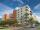The residential house has three entrances numbered even numbers, successive immediately behind. The sum of the two numbers on the outside entrances is 68. Calculate the middle of these three numbers.
7. TogetherIf 8 men, 10 women, 16 children collects ₹1024 in 4 days, how many days will be required for 6 men, 5 women and 4 boys to collect ₹768? (₹ is Indian Rupee)
8. Triangles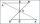Hanka cut the 20 cm long straws into three pieces each piece had a length in cm. Then, with these three pieces, she tried to make a triangle. a) What circuit has each of the triangles? b) How long can the longest side measure? c) How many different tria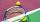The children of the tennis school received 64 white and 48 yellow balls from the sponsor. When asked about how many balls they could take, they were answered: "You have so many that none of you will have more than 10 balls and all will have the same numbe
10. TrianglesIvo wants to draw all the triangles whose two sides of which have a length of 4 cm and 9 cm and the length of the third side is expressed in whole centimeters. How many triangles does he have?
11. Mysterious number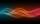The magician thinks the number: "The mysterious number is first divided by minus five, dividing the result by three, multiplying the number by ten, and dividing the resulting number by minus four. This gives result 5. Can you reveal the mysterious number
12. Simple sequence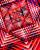Continue with this series of numbers: 1792,448, 112, _, _
13. Dinosaurs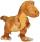More than 30 and less than 60 dinosaurs have met at the pond. A quarter of them bathed and 1/7 saws and the rest gripped. How many were at the pond? How many were there?
14. Twenty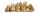Twenty rabbits are put in 4 cells so that there are different number of rabbits in each cell contains at least 3 rabbits. What is the largest possible number of rabbits in one cell
15. A numberA number increased by 7.9 is 8.3
16. Find twoFind two consecutive natural numbers whose product is 1 larger than their sum. Searched numbers expressed by a fraction whose numerator is the difference between these numbers and the denominator is their sum.
17. Two numbers 6Fill two natural numbers a, b: 7 + blank- blank = 5
18. I thinkI think a number. When I multiply it by five, and after that I subtract 477, I get the same number as if I multiplied it twice. What number do I think?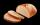They brought in d two kilos and t three kilos of bread to the store. By the end of the day, 14 two-kg bread were sold. How many kilos of bread remained in the store by the next day?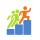Peter walked a distance of 60 m 102 steps. How many steps must he take to walk 150 m?